﻿ 考虑用户异质性和瓶颈处停车换乘的交通定价模型研究 The Pricing Model of Parking and Transit Fare Considering Park-and-Ride Facilities and User Heterogeneity

Management Science and Engineering
Vol. 08  No. 04 ( 2019 ), Article ID: 32903 , 12 pages
10.12677/MSE.2019.84034

The Pricing Model of Parking and Transit Fare Considering Park-and-Ride Facilities and User Heterogeneity

Luyi Yu, Xiaoning Zhang

School of Economics and Management, Tongji University, Shanghai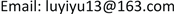Received: Oct. 16th, 2019; accepted: Nov. 4th, 2019; published: Nov. 11th, 2019ABSTRACT

This paper extends the bottleneck model and develops a bi-level programming model to study the traffic charge problem between two companies, one of which operates buses and park-and-ride (P&R) parking lots simultaneously, while the other of which owns central parking lots. Therefore, commuters can get their destination by either auto or P&R mode. The proposed model analyzes two game scenarios: perfect competition and market regulation and considers heterogeneous users who are distinguished by their valuation of travel time. Specifically, in the upper level, the objective of operators is to maximize their own net profit; in the lower level, the followers would choose the optimal travel plans given the traffic charge rules. Finally, numerical examples are provided to illustrate the effectiveness of the developed models; furthermore, the result shows that when the proportion of commuters with higher value of time (VOT) is increasing, the gap of total social costs between two game scenarios becomes larger.

Keywords:Park-and-Ride, User Heterogeneity, Traffic Charge, Bi-Level Programming, Bottleneck Model1. 引言

2. 模型建立

2.1. 模型背景及参数说明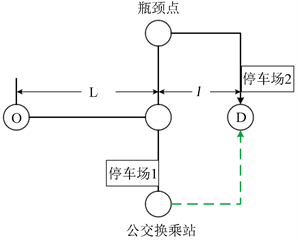Figure 1. A one-to-one bi-modal transportation system

$\begin{array}{l}{\alpha }_{w}>{\alpha }_{b},\text{}{\beta }_{w}<{\beta }_{b},\text{}\frac{{\gamma }_{w}}{{\beta }_{w}}=\frac{{\gamma }_{b}}{{\beta }_{b}}=\eta \\ {\theta }_{w}>{\theta }_{b}\\ {\alpha }_{w}/{\theta }_{w}>{\alpha }_{b}/{\theta }_{b}\end{array}$ (1)Table 1. List of other notations involved in the model

2.2. 上层交通定价优化目标

$\mathrm{max}T{R}_{P}={p}_{2}{N}^{A}-{c}_{2}N$ (2)

$\mathrm{max}T{R}_{Q}=\left({p}_{1}+P\left(l\right)-a\right){N}^{T}-{c}_{1}N-F$ (3)

$\mathrm{max}TR={p}_{2}{N}^{A}+\left({p}_{1}+P\left(l\right)\right){N}^{T}-a{N}^{T}-\underset{i=w,b}{\sum }\left[{N}_{i}^{A}\left(M{C}_{i}^{A}\right)+{N}_{i}^{T}\left(M{C}_{i}^{T}\right)\right]-\left({c}_{1}+{c}_{2}\right)N-F$ (4)

2.3. 下层交通出行广义出行费用

2.3.1. 全程自驾出行费用

${C}_{i}^{A}\left(t\right)={\alpha }_{i}T\left(t\right)+{\beta }_{i}\mathrm{max}\left\{0,{t}^{*}-t-T\left(t\right)\right\}+{\gamma }_{i}\mathrm{max}\left\{0,t+T\left(t\right)-{t}^{*}\right\}+{p}_{2},\text{}i=w,b$ (5)

2.3.2. P&R出行费用

${C}_{i}^{T}\left(l\right)={\alpha }_{i}\left(\frac{L}{{v}_{1}}+\frac{l}{{v}_{2}}+T\right)+{\theta }_{i}{N}^{T}+P\left(l\right)+{p}_{1}\text{,}i=w,b$ (6)

3. 用户出行均衡状态分析

3.1. 人均交通出行成本分析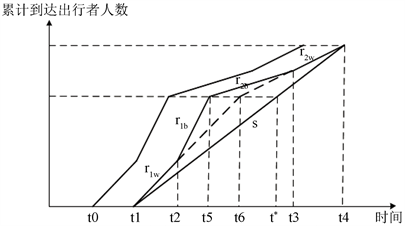Figure 2. The auto commuting equilibrium traffic pattern

$M{C}_{w}^{A}=\frac{{\delta }_{w}{N}^{A}}{s}+{\alpha }_{w}\frac{L+l}{{v}_{1}}+{p}_{2}$ (7)

$M{C}_{b}^{A}=\frac{{\delta }_{b}{N}_{b}^{A}}{s}+\frac{{\alpha }_{b}}{{\alpha }_{w}}\frac{{\delta }_{w}{N}_{w}^{A}}{s}+{\alpha }_{b}\frac{L+l}{{v}_{1}}+{p}_{2}$ (8)

$M{C}_{w}^{T}={\alpha }_{w}\left(\frac{L}{{v}_{1}}+\frac{l}{{v}_{2}}+T\right)+{\theta }_{w}{N}^{T}+P\left(l\right)+{p}_{1}$ (9)

$M{C}_{b}^{T}={\alpha }_{b}\left(\frac{L}{{v}_{1}}+\frac{l}{{v}_{2}}+T\right)+{\theta }_{b}{N}^{T}+P\left(l\right)+{p}_{1}$ (10)

3.2. 交通出行模式组合概览

${N}^{A}=\frac{{\alpha }_{w}s\left(\frac{l}{{v}_{2}}-\frac{l}{{v}_{1}}+T\right)+\left(P\left(l\right)+{p}_{1}-{p}_{2}\right)s+{\theta }_{w}sN}{{\delta }_{w}+s{\theta }_{w}}$ (11)

${N}^{T}=\frac{{\delta }_{w}N-{\alpha }_{w}s\left(\frac{l}{{v}_{2}}-\frac{l}{{v}_{1}}+T\right)-\left(P\left(l\right)+{p}_{1}-{p}_{2}\right)s}{{\delta }_{w}+s{\theta }_{w}}$ (12)

4. 交通定价策略优化分析

4.1. 企业P和企业Q净利润最大(自由竞争模式)

$\mathrm{max}T{R}_{P}=\frac{-{\left({p}_{2}\right)}^{2}s+{p}_{2}\left[{\alpha }_{w}s\left(\frac{l}{{v}_{2}}-\frac{l}{{v}_{1}}+T\right)+\left(P\left(l\right)+{p}_{1}\right)s+{\theta }_{w}sN\right]}{{\delta }_{w}+s{\theta }_{w}}-{c}_{2}N$ (13)

$\begin{array}{l}\mathrm{max}T{R}_{Q}=\frac{-{\left(P\left(l\right)+{p}_{1}\right)}^{2}s+\left(P\left(l\right)+{p}_{1}\right)\left[{\delta }_{w}N-{\alpha }_{w}s\left(\frac{l}{{v}_{2}}-\frac{l}{{v}_{1}}+T\right)+{p}_{2}s+as\right]}{{\delta }_{w}+s{\theta }_{w}}\\ \text{}\text{\hspace{0.17em}}\text{\hspace{0.17em}}\text{\hspace{0.17em}}-\left[a\frac{{\delta }_{w}N-{\alpha }_{w}s\left(\frac{l}{{v}_{2}}-\frac{l}{{v}_{1}}+T\right)+{p}_{2}s}{{\delta }_{w}+s{\theta }_{w}}+{c}_{1}N+F\right]\end{array}$ (14)

${p}_{2}=\frac{P\left(l\right)+{p}_{1}+{\alpha }_{w}\left(\frac{l}{{v}_{2}}-\frac{l}{{v}_{1}}+T\right)+{\theta }_{w}N}{2}$ (15)

$P\left(l\right)+{p}_{1}=\frac{1}{2}\left[\frac{{\delta }_{w}N}{s}-{\alpha }_{w}\left(\frac{l}{{v}_{2}}-\frac{l}{{v}_{1}}+T\right)+{p}_{2}+a\right]$ (16)

${p}_{2}=\frac{2{\theta }_{w}N}{3}+\frac{1}{3}\left(\frac{{\delta }_{w}N}{s}+a-{\alpha }_{w}\left(\frac{l}{{v}_{2}}-\frac{l}{{v}_{1}}+T\right)\right)$ (17)

$P\left(l\right)+{p}_{1}=\frac{1}{3}\left[{\theta }_{w}N-{\alpha }_{w}\left(\frac{l}{{v}_{2}}-\frac{l}{{v}_{1}}+T\right)\right]+\frac{2}{3}\left(\frac{{\delta }_{w}N}{s}+a\right)$ (18)

4.2. 社会净收益最大(政府干预模式)

$\begin{array}{l}\mathrm{min}TC={N}_{w}^{A}\left(\frac{{\delta }_{w}{N}^{A}}{s}+{\alpha }_{w}\frac{L+l}{{v}_{1}}\right)+{N}_{b}^{A}\left(\frac{{\delta }_{b}{N}_{b}^{A}}{s}+\frac{{\alpha }_{b}}{{\alpha }_{w}}\frac{{\delta }_{w}{N}_{w}^{A}}{s}+{\alpha }_{b}\frac{L+l}{{v}_{1}}\right)\\ \text{}+{N}_{w}^{T}\left[{\alpha }_{w}\left(\frac{L}{{v}_{1}}+\frac{l}{{v}_{2}}+T\right)+{\theta }_{w}{N}^{T}\right]+{N}_{b}^{T}\left[{\alpha }_{b}\left(\frac{L}{{v}_{1}}+\frac{l}{{v}_{2}}+T\right)+{\theta }_{b}{N}^{T}\right]\\ \text{}+a{N}^{T}+F+\left({c}_{1}+{c}_{2}\right)N\end{array}$ (19)

$\begin{array}{l}\mathrm{min}TC=\left({\alpha }_{w}{N}_{w}+{\alpha }_{b}{N}_{b}\right)\left(\frac{L}{{v}_{1}}+\frac{l}{{v}_{2}}+T\right)+{N}^{T}\left({\theta }_{w}{N}_{w}+{\theta }_{b}{N}_{b}\right)\\ \text{}+\left(N-{N}^{T}\right)\left(P\left(l\right)+{p}_{1}-{p}_{2}\right)+a{N}^{T}+F+\left({c}_{1}+{c}_{2}\right)N\end{array}$ (20)

$P\left(l\right)+{p}_{1}-{p}_{2}=\frac{a-\left[{N}_{b}\left({\theta }_{w}-{\theta }_{b}\right)+{\alpha }_{w}\left(\frac{l}{{v}_{2}}-\frac{l}{{v}_{1}}+T\right)\right]}{2}$ (21)

${N}^{A}=\frac{as+{N}_{b}s\left({\theta }_{w}+{\theta }_{b}\right)+{\alpha }_{w}s\left(\frac{l}{{v}_{2}}-\frac{l}{{v}_{1}}+T\right)+2{\theta }_{w}s{N}_{w}}{2\left({\delta }_{w}+s{\theta }_{w}\right)}$ (22)

${N}^{T}=\frac{2{\delta }_{w}N-{\alpha }_{w}s\left(\frac{l}{{v}_{2}}-\frac{l}{{v}_{1}}+T\right)-as+{N}_{b}s\left({\theta }_{w}-{\theta }_{b}\right)}{{\delta }_{w}+s{\theta }_{w}}$ (23)

5. 算例分析

5.1. 停车换乘时间和通行能力变化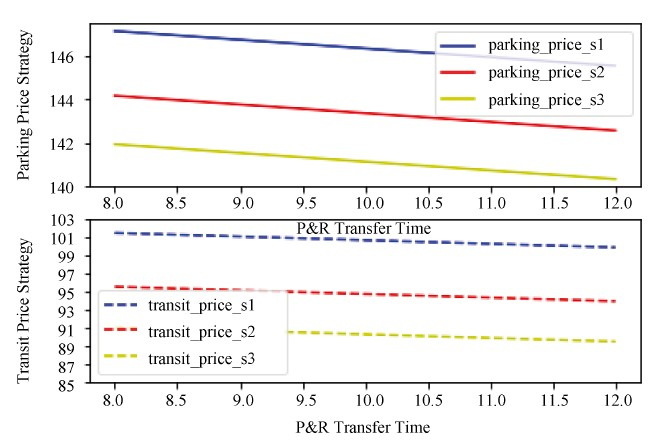Figure 3. The prices of company p and company Q versus T with different s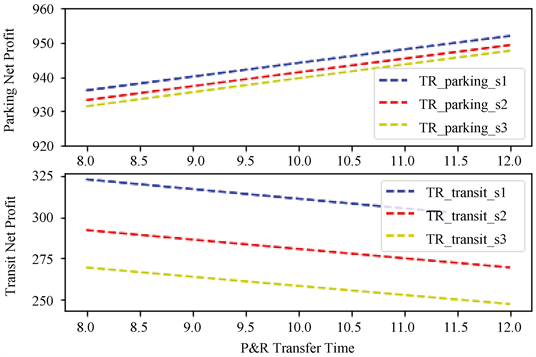Figure 4. The net profit of company p and company Q versus T with different s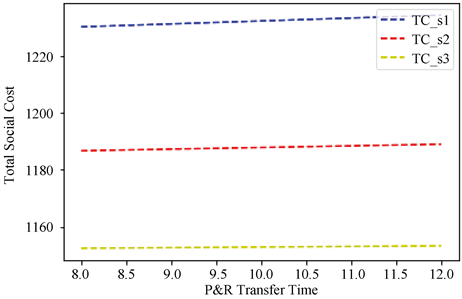Figure 5. The total social cost versus T with different s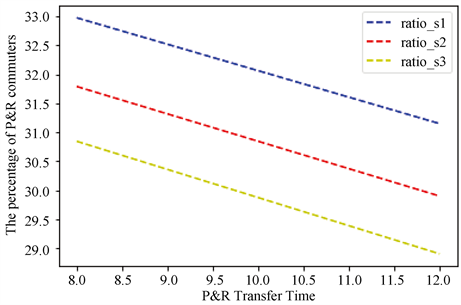Figure 6. The percentage of P&R versus T with different s

5.2. 两类出行者所占比例对系统总成本的影响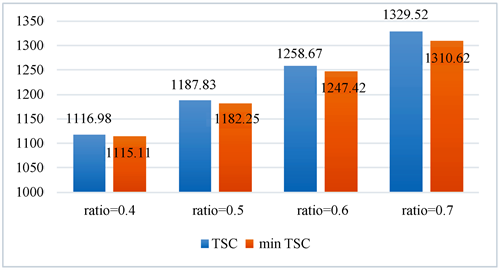Figure 7. The total cost with or without government intervention versus different Nw

6. 结语

The Pricing Model of Parking and Transit Fare Considering Park-and-Ride Facilities and User Heterogeneity[J]. 管理科学与工程, 2019, 08(04): 285-296. https://doi.org/10.12677/MSE.2019.84034

1. 1. Zhao, X.W., Chen, P., Jiao, J.F., et al. (2019) How Does “Park and Ride” Perform? An Evaluation Using Longitudinal Data. Transport Policy, 74, 15-23.
https://doi.org/10.1016/j.tranpol.2018.11.004

2. 2. 李玉涛, 周江评. 重新定位停车政策:优化出行的重要工具[J]. 城市规划, 2015(4): 71-75.

3. 3. Qian, Z.S. and Rajagopal, R. (2014) Optimal Occupancy-Driven Parking Pricing under Demand Uncertainties and Traveler Heterogeneity: A Stochastic Control Approach. Transportation Research Part B: Methodological, 67, 144-165.
https://doi.org/10.1016/j.trb.2014.03.002

4. 4. Liu, Z.Y., Chen, X.Y., Meng, Q. and Inhi, K. (2018) Remote Park-and-Ride Network Equilibrium Model and Its Applications. Transportation Research Part B: Methodological, 117, 37-62.
https://doi.org/10.1016/j.trb.2018.08.004

5. 5. Mills, G. and White, P. (2018) Evaluating the Long-Term Impacts of Bus-Based Park and Ride. Research in Transportation Economics, 69, 536-543.
https://doi.org/10.1016/j.retrec.2018.07.028

6. 6. 喻军皓. 上海市P+R停车场库发展策略研究[C]//中国城市规划设计研究院城市交通专业研究院. 创新驱动与智慧发展——2018年中国城市交通规划年会论文集. 2018: 15.

7. 7. Song, Z., He, Y. and Zhang, L. (2017) Integrated Planning of Park-and-Ride Facilities and Transit Service. Transportation Research Part C: Emerging Technologies, 74, 182-195.
https://doi.org/10.1016/j.trc.2016.11.017

8. 8. Aros-Vera, F., Marianov, V. and Mitchell, J.E. (2013) P-Hub Approach for the Optimal Park-and-Ride Facility Location Problem. European Journal of Operational Research, 226, 277-285.
https://doi.org/10.1016/j.ejor.2012.11.006

9. 9. Holguın-Veras, J., Yushimito, W.F., Aros-Vera, F., et al. (2012) User Rationality and Optimal Park-and-Ride Location under Potential Demand Maximization. Transportation Research Part B: Methodological, 46, 949-970.
https://doi.org/10.1016/j.trb.2012.02.011

10. 10. 田琼, 黄海军, 杨海. 瓶颈处停车换乘logit随机均衡选择模型[J]. 管理科学学报, 2005, 8(1): 1-6.

11. 11. Liu, Z., Meng, Q., et al. (2014) Bus-Based Park-and-Ride System: A Stochastic Model on Multimodal Network with Congestion Pricing Schemes. International Journal of Systems Science, 45, 994-1006.
https://doi.org/10.1080/00207721.2012.743617

12. 12. Gao, G., Sun, H., Wu, J., et al. (2018) Park-and-Ride Service Design under a Price-Based Tradable Credits Scheme in a Linear Monocentric City. Transport Policy, 68, 1-12.
https://doi.org/10.1016/j.tranpol.2018.04.001

13. 13. Cohen, Y. (1987) Commuter Welfare under Peak-Period Congestion Tolls: Who Gains and Who Loses. International Journal of Transport Economics, 14, 239-266.

14. 14. Arnott, R., Palma, A.D. and Lindsey, R. (1993) The Welfare Effects of Congestion Tolls with Heterogeneous Commuters. Boston College Working Papers in Economics, 28, 139-161.

15. 15. Liu, Y. and Nie, Y. (2011) Morning Commute Problem Considering Route Choice, User Heterogeneity and Alternative System Optima. Transportation Research Part B Methodological, 45, 619-642.
https://doi.org/10.1016/j.trb.2010.11.003

16. 16. 朱成娟. 考虑停车换乘的多方式交通网络出行行为分析[D]: [博士学位论文]. 北京: 北京交通大学, 2015.